This book is archived and will be removed July 6, 2022. Please use the updated version.

Quantum Mechanics

# 54 The Quantum Harmonic Oscillator

### Learning Objectives

By the end of this section, you will be able to:

• Describe the model of the quantum harmonic oscillator
• Identify differences between the classical and quantum models of the harmonic oscillator
• Explain physical situations where the classical and the quantum models coincide

Oscillations are found throughout nature, in such things as electromagnetic waves, vibrating molecules, and the gentle back-and-forth sway of a tree branch. In previous chapters, we used Newtonian mechanics to study macroscopic oscillations, such as a block on a spring and a simple pendulum. In this chapter, we begin to study oscillating systems using quantum mechanics. We begin with a review of the classic harmonic oscillator.

### The Classic Harmonic Oscillator

A simple harmonic oscillator is a particle or system that undergoes harmonic motion about an equilibrium position, such as an object with mass vibrating on a spring. In this section, we consider oscillations in one-dimension only. Suppose a mass moves back-and-forth along the

x-direction about the equilibrium position,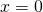. In classical mechanics, the particle moves in response to a linear restoring force given by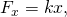where x is the displacement of the particle from its equilibrium position. The motion takes place between two turning points,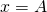, where A denotes the amplitude of the motion. The position of the object varies periodically in time with angular frequency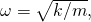which depends on the mass m of the oscillator and on the force constant k of the net force, and can be written as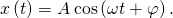The total energy E of an oscillator is the sum of its kinetic energy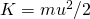and the elastic potential energy of the force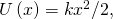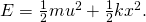At turning points, the speed of the oscillator is zero; therefore, at these points, the energy of oscillation is solely in the form of potential energy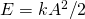. The plot of the potential energy U(x) of the oscillator versus its position x is a parabola ((Figure)). The potential-energy function is a quadratic function of x, measured with respect to the equilibrium position. On the same graph, we also plot the total energy E of the oscillator, as a horizontal line that intercepts the parabola at. Then the kinetic energy K is represented as the vertical distance between the line of total energy and the potential energy parabola.

The potential energy well of a classical harmonic oscillator: The motion is confined between turning points at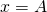and at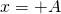. The energy of oscillations is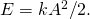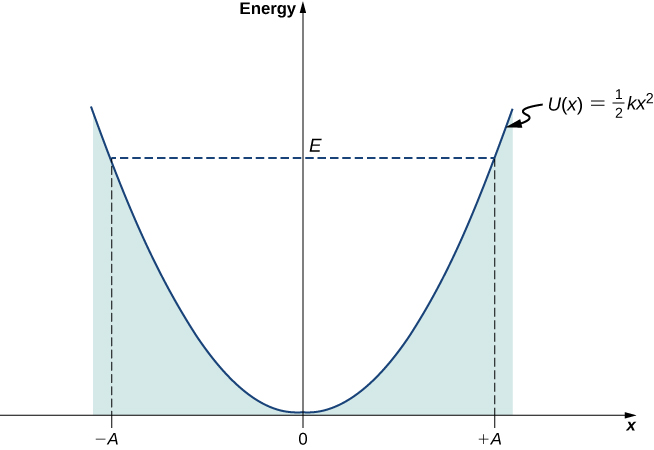In this plot, the motion of a classical oscillator is confined to the region where its kinetic energy is nonnegative, which is what the energy relation (Figure) says. Physically, it means that a classical oscillator can never be found beyond its turning points, and its energy depends only on how far the turning points are from its equilibrium position. The energy of a classical oscillator changes in a continuous way. The lowest energy that a classical oscillator may have is zero, which corresponds to a situation where an object is at rest at its equilibrium position. The zero-energy state of a classical oscillator simply means no oscillations and no motion at all (a classical particle sitting at the bottom of the potential well in (Figure)). When an object oscillates, no matter how big or small its energy may be, it spends the longest time near the turning points, because this is where it slows down and reverses its direction of motion. Therefore, the probability of finding a classical oscillator between the turning points is highest near the turning points and lowest at the equilibrium position. (Note that this is not a statement of preference of the object to go to lower energy. It is a statement about how quickly the object moves through various regions.)

### The Quantum Harmonic Oscillator

One problem with this classical formulation is that it is not general. We cannot use it, for example, to describe vibrations of diatomic molecules, where quantum effects are important. A first step toward a quantum formulation is to use the classical expression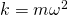to limit mention of a “spring” constant between the atoms. In this way the potential energy function can be written in a more general form,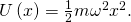Combining this expression with the time-independent Schrӧdinger equation gives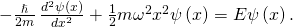To solve (Figure)—that is, to find the allowed energies E and their corresponding wave functions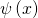—we require the wave functions to be symmetric about(the bottom of the potential well) and to be normalizable. These conditions ensure that the probability densitymust be finite when integrated over the entire range of x from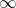to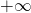. How to solve (Figure) is the subject of a more advanced course in quantum mechanics; here, we simply cite the results. The allowed energies are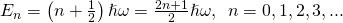The wave functions that correspond to these energies (the stationary states or states of definite energy) are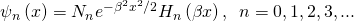where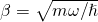,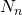is the normalization constant, and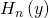is a polynomial of degree n called a Hermite polynomial. The first four Hermite polynomials are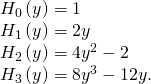A few sample wave functions are given in (Figure). As the value of the principal number increases, the solutions alternate between even functions and odd functions about.

The first five wave functions of the quantum harmonic oscillator. The classical limits of the oscillator’s motion are indicated by vertical lines, corresponding to the classical turning points atof a classical particle with the same energy as the energy of a quantum oscillator in the state indicated in the figure.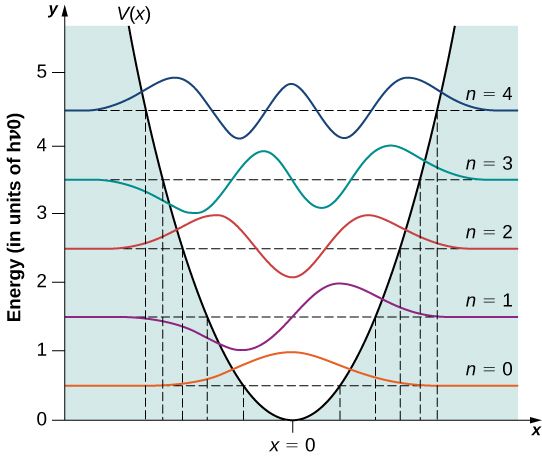Classical Region of Harmonic Oscillations Find the amplitude A of oscillations for a classical oscillator with energy equal to the energy of a quantum oscillator in the quantum state n.

Strategy To determine the amplitude A, we set the classical energy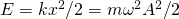equal to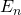given by (Figure).

Solution We obtain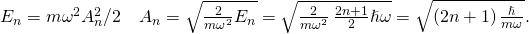Significance As the quantum number n increases, the energy of the oscillator and therefore the amplitude of oscillation increases (for a fixed natural angular frequency. For large n, the amplitude is approximately proportional to the square root of the quantum number.

Several interesting features appear in this solution. Unlike a classical oscillator, the measured energies of a quantum oscillator can have only energy values given by (Figure). Moreover, unlike the case for a quantum particle in a box, the allowable energy levels are evenly spaced,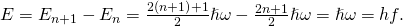When a particle bound to such a system makes a transition from a higher-energy state to a lower-energy state, the smallest-energy quantum carried by the emitted photon is necessarily hf. Similarly, when the particle makes a transition from a lower-energy state to a higher-energy state, the smallest-energy quantum that can be absorbed by the particle is hf. A quantum oscillator can absorb or emit energy only in multiples of this smallest-energy quantum. This is consistent with Planck’s hypothesis for the energy exchanges between radiation and the cavity walls in the blackbody radiation problem.

Vibrational Energies of the Hydrogen Chloride MoleculeThe HCl diatomic molecule consists of one chlorine atom and one hydrogen atom. Because the chlorine atom is 35 times more massive than the hydrogen atom, the vibrations of the HCl molecule can be quite well approximated by assuming that the Cl atom is motionless and the H atom performs harmonic oscillations due to an elastic molecular force modeled by Hooke’s law. The infrared vibrational spectrum measured for hydrogen chloride has the lowest-frequency line centered at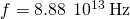. What is the spacing between the vibrational energies of this molecule? What is the force constant k of the atomic bond in the HCl molecule?

Strategy The lowest-frequency line corresponds to the emission of lowest-frequency photons. These photons are emitted when the molecule makes a transition between two adjacent vibrational energy levels. Assuming that energy levels are equally spaced, we use (Figure) to estimate the spacing. The molecule is well approximated by treating the Cl atom as being infinitely heavy and the H atom as the mass m that performs the oscillations. Treating this molecular system as a classical oscillator, the force constant is found from the classical relation.

Solution The energy spacing is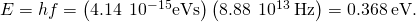The force constant is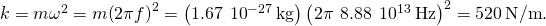Significance The force between atoms in an HCl molecule is surprisingly strong. The typical energy released in energy transitions between vibrational levels is in the infrared range. As we will see later, transitions in between vibrational energy levels of a diatomic molecule often accompany transitions between rotational energy levels.

Check Your Understanding The vibrational frequency of the hydrogen iodide HI diatomic molecule is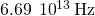. (a) What is the force constant of the molecular bond between the hydrogen and the iodine atoms? (b) What is the energy of the emitted photon when this molecule makes a transition between adjacent vibrational energy levels?

a. 295 N/m; b. 0.277 eV

The quantum oscillator differs from the classic oscillator in three ways:

First, the ground state of a quantum oscillator is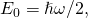not zero. In the classical view, the lowest energy is zero. The nonexistence of a zero-energy state is common for all quantum-mechanical systems because of omnipresent fluctuations that are a consequence of the Heisenberg uncertainty principle. If a quantum particle sat motionless at the bottom of the potential well, its momentum as well as its position would have to be simultaneously exact, which would violate the Heisenberg uncertainty principle. Therefore, the lowest-energy state must be characterized by uncertainties in momentum and in position, so the ground state of a quantum particle must lie above the bottom of the potential well.

Second, a particle in a quantum harmonic oscillator potential can be found with nonzero probability outside the interval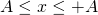. In a classic formulation of the problem, the particle would not have any energy to be in this region. The probability of finding a ground-state quantum particle in the classically forbidden region is about 16%.

Third, the probability density distributions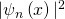for a quantum oscillator in the ground low-energy state,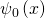, is largest at the middle of the well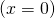. For the particle to be found with greatest probability at the center of the well, we expect that the particle spends the most time there as it oscillates. This is opposite to the behavior of a classical oscillator, in which the particle spends most of its time moving with relative small speeds near the turning points.

Check Your Understanding Find the expectation value of the position for a particle in the ground state of a harmonic oscillator using symmetry.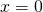Quantum probability density distributions change in character for excited states, becoming more like the classical distribution when the quantum number gets higher. We observe this change already for the first excited state of a quantum oscillator because the distribution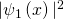peaks up around the turning points and vanishes at the equilibrium position, as seen in (Figure). In accordance with Bohr’s correspondence principle, in the limit of high quantum numbers, the quantum description of a harmonic oscillator converges to the classical description, which is illustrated in (Figure). The classical probability density distribution corresponding to the quantum energy of the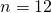state is a reasonably good approximation of the quantum probability distribution for a quantum oscillator in this excited state. This agreement becomes increasingly better for highly excited states.

The probability density distribution for finding the quantum harmonic oscillator in itsquantum state. The dashed curve shows the probability density distribution of a classical oscillator with the same energy.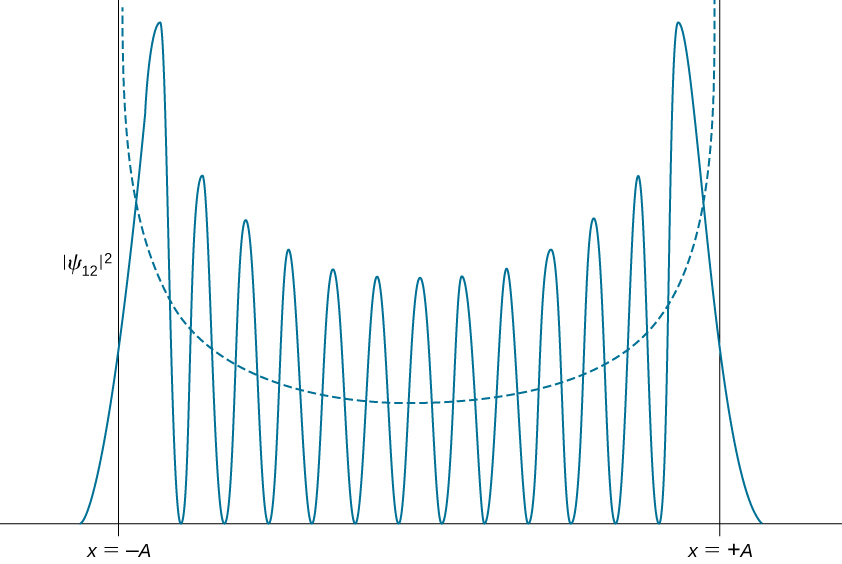### Summary

• The quantum harmonic oscillator is a model built in analogy with the model of a classical harmonic oscillator. It models the behavior of many physical systems, such as molecular vibrations or wave packets in quantum optics.
• The allowed energies of a quantum oscillator are discrete and evenly spaced. The energy spacing is equal to Planck’s energy quantum.
• The ground state energy is larger than zero. This means that, unlike a classical oscillator, a quantum oscillator is never at rest, even at the bottom of a potential well, and undergoes quantum fluctuations.
• The stationary states (states of definite energy) have nonzero values also in regions beyond classical turning points. When in the ground state, a quantum oscillator is most likely to be found around the position of the minimum of the potential well, which is the least-likely position for a classical oscillator.
• For high quantum numbers, the motion of a quantum oscillator becomes more similar to the motion of a classical oscillator, in accordance with Bohr’s correspondence principle.

### Conceptual Questions

Is it possible to measure energy of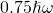for a quantum harmonic oscillator? Why? Why not? Explain.

No. This energy corresponds to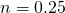, but n must be an integer.

Explain the connection between Planck’s hypothesis of energy quanta and the energies of the quantum harmonic oscillator.

If a classical harmonic oscillator can be at rest, why can the quantum harmonic oscillator never be at rest? Does this violate Bohr’s correspondence principle?

Because the smallest allowed value of the quantum number n for a simple harmonic oscillator is 0. No, because quantum mechanics and classical mechanics agree only in the limit of large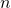.

Use an example of a quantum particle in a box or a quantum oscillator to explain the physical meaning of Bohr’s correspondence principle.

Can we simultaneously measure position and energy of a quantum oscillator? Why? Why not?

Yes, within the constraints of the uncertainty principle. If the oscillating particle is localized, the momentum and therefore energy of the oscillator are distributed.

### Problems

Show that the two lowest energy states of the simple harmonic oscillator,and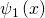from (Figure), satisfy (Figure).

proof

If the ground state energy of a simple harmonic oscillator is 1.25 eV, what is the frequency of its motion?

When a quantum harmonic oscillator makes a transition from the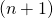state to the n state and emits a 450-nm photon, what is its frequency?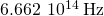Vibrations of the hydrogen molecule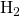can be modeled as a simple harmonic oscillator with the spring constant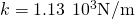and mass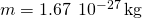. (a) What is the vibrational frequency of this molecule? (b) What are the energy and the wavelength of the emitted photon when the molecule makes transition between its third and second excited states?

A particle with mass 0.030 kg oscillates back-and-forth on a spring with frequency 4.0 Hz. At the equilibrium position, it has a speed of 0.60 m/s. If the particle is in a state of definite energy, find its energy quantum number.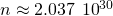Find the expectation value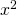of the square of the position for a quantum harmonic oscillator in the ground state. Note: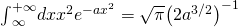.

Determine the expectation value of the potential energy for a quantum harmonic oscillator in the ground state. Use this to calculate the expectation value of the kinetic energy.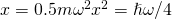;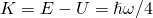Verify thatgiven by (Figure) is a solution of Schrӧdinger’s equation for the quantum harmonic oscillator.

Estimate the ground state energy of the quantum harmonic oscillator by Heisenberg’s uncertainty principle. Start by assuming that the product of the uncertainties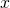and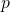is at its minimum. Writein terms ofand assume that for the ground state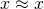and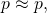then write the ground state energy in terms of x. Finally, find the value of x that minimizes the energy and find the minimum of the energy.

proof

A mass of 0.250 kg oscillates on a spring with the force constant 110 N/m. Calculate the ground energy level and the separation between the adjacent energy levels. Express the results in joules and in electron-volts. Are quantum effects important?